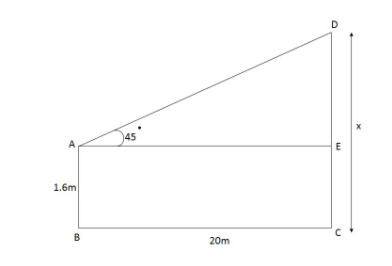QuestionAnswers

# A boy 1.6 m tall is 20 m away from a tower and observes the angle of elevation of the top of tower to be (i) 45° (ii) 60°Find the height of the tower in each case.

Hint: When a boy stands near a tower and observes its top it makes a right triangle with angle 45° and 60°using trigonometric formula we can find height.

Let the height of the tower CD be x
Height of boy (AB) = CE
So, height of DE = CD – CE
= x – 1.6In the triangle ΔDAE
∠DAE = 45°
From the trigonometric formula
$\tan \theta = \dfrac{{perpendicular}}{{base}}$
∵ Distance between the boy and tower
= 20 cm
So, BC = 20 cm
tan 45 $= \dfrac{{x - 1.6}}{{20}}$
1 × 20 = x – 1.6 (∵ tan 45° = 1)
x = 21.6
tan 60° $= \dfrac{{x - 1.6}}{{20}}$
$20\sqrt 3 + 1.6 = x$
x = 36.24
So, the height of tower in case of elevation of 45° = 21.6
And the height of tower in case of elevation of 60° = 36.24.

Note: In this type of problem first try to draw a rough diagram. Mark the angle which is given in question. Using trigonometric formulas we can find the side length and also we can find angle if length is given in the question.